# parrt/dtreeviz

A python library for decision tree visualization and model interpretation.
Latest commit 3212e26 Jul 15, 2019
Type Name Latest commit message Commit time
Failed to load latest commit information..idea Jun 13, 2019dtreeviz Jul 6, 2019notebooks Jul 14, 2019talk Jan 10, 2019testing Jul 14, 2019.gitignore Aug 13, 2018LICENSE Aug 13, 2018README.md Jul 6, 2019play.ipynb Oct 2, 2018setup.cfg Sep 29, 2018setup.py Jul 5, 2019

# dtreeviz : Decision Tree Visualization

## Description

A python library for decision tree visualization and model interpretation.

See How to visualize decision trees for deeper discussion of our decision tree visualization library and the visual design decisions we made.

## Discussion

Decision trees are the fundamental building block of gradient boosting machines and Random Forests(tm), probably the two most popular machine learning models for structured data. Visualizing decision trees is a tremendous aid when learning how these models work and when interpreting models. Unfortunately, current visualization packages are rudimentary and not immediately helpful to the novice. For example, we couldn't find a library that visualizes how decision nodes split up the feature space. It is also uncommon for libraries to support visualizing a specific feature vector as it weaves down through a tree's decision nodes; we could only find one image showing this.

So, we've created a general package for scikit-learn decision tree visualization and model interpretation, which we'll be using heavily in an upcoming machine learning book (written with Jeremy Howard).

The visualizations are inspired by an educational animation by R2D3; A visual introduction to machine learning. With dtreeviz, you can visualize how the feature space is split up at decision nodes, how the training samples get distributed in leaf nodes and how the tree makes predictions for a specific observation. These operations are critical to for understanding how classification or regression decision trees work. If you're not familiar with decision trees, check out fast.ai's Introduction to Machine Learning for Coders MOOC.

## Install

You might verify that you do not have conda-installed graphviz-related packages installed because dtreeviz needs the pip versions; you can remove them from conda space by doing:

conda uninstall python-graphviz
conda uninstall graphviz

To install (Python >=3.6 only), do this (from Anaconda Prompt on Windows!):

pip install dtreeviz

This should also pull in the graphviz Python library (>=0.9), which we are using for platform specific stuff.

Limitations. Only svg files can be generated at this time, which reduces dependencies and dramatically simplifies install process.

Please email Terence with any helpful notes on making dtreeviz work (better) on other platforms. Thanks!

### Mac

Make sure to have the latest XCode installed and command-line tools installed. You can run xcode-select --install from the command-line to install those if XCode is already installed. You also have to sign the XCode license agreement, which you can do with sudo xcodebuild -license from command-line. The brew install shown next needs to build graphviz, so you need XCode set up properly.

You need the graphviz binary for dot. Make sure you have latest version (verified on 10.13, 10.14):

brew reinstall graphviz

Just to be sure, remove dot from any anaconda installation, for example:

rm ~/anaconda3/bin/dot

From command line, this command

dot -Tsvg

should work, in the sense that it just stares at you without giving an error. You can hit control-C to escape back to the shell. Make sure that you are using the right dot as installed by brew:

$which dot /usr/local/bin/dot$ ls -l $(which dot) lrwxr-xr-x 1 parrt wheel 33 May 26 11:04 /usr/local/bin/dot@ -> ../Cellar/graphviz/2.40.1/bin/dot$

Limitations. Jupyter notebook has a bug where they do not show .svg files correctly, but Juypter Lab has no problem.

### Linux (Ubuntu 18.04)

To get the dot binary do:

sudo apt install graphviz

Limitations. The view() method works to pop up a new window and images appear inline for jupyter notebook but not jupyter lab (It gets an error parsing the SVG XML.) The notebook images also have a font substitution from the Arial we use and so some text overlaps. Only .svg files can be generated on this platform.

### Windows 10

Download graphviz-2.38.msi and update your Path environment variable. It's windows so you might need a reboot after updating that environment variable. You should see this from the Anaconda Prompt:

(base) C:\Users\Terence Parr>where dot
C:\Program Files (x86)\Graphviz2.38\bin\dot.exe


(Do not use conda install -c conda-forge python-graphviz as you get an old version of graphviz python library.)

Verify from the Anaconda Prompt that this works (capital -V not lowercase -v):

dot -V


If it doesn't work, you have a Path problem. I found the following test programs useful. The first one sees if Python can find dot:

import os
import subprocess
proc = subprocess.Popen(['dot','-V'])
print( os.getenv('Path') )

The following version does the same thing except uses graphviz Python libraries backend support utilities, which is what we use in dtreeviz:

import graphviz.backend as be
cmd = ["dot", "-V"]
stdout, stderr = be.run(cmd, capture_output=True, check=True, quiet=False)
print( stderr )

If you are having issues with run command you can try copying the following files from: https://github.com/xflr6/graphviz/tree/master/graphviz.

Place them in the AppData\Local\Continuum\anaconda3\Lib\site-packages\graphviz folder.

Clean out the pycache directory too.

Jupyter Lab and Jupyter notebook both show the inline .svg images well.

### Verify graphviz installation

Try making text file t.dot with content digraph T { A -> B } (paste that into a text editor, for example) and then running this from the command line:

dot -Tsvg -o t.svg t.dot


That should give a simple t.svg file that opens properly. If you get errors from dot, it will not work from the dtreeviz python code. If it can't find dot then you didn't update your PATH environment variable or there is some other install issue with graphviz.

### Limitations

Finally, don't use IE to view .svg files. Use Edge as they look much better. I suspect that IE is displaying them as a rasterized not vector images. Only .svg files can be generated on this platform.

## Usage

dtree: Main function to create decision tree visualization. Given a decision tree regressor or classifier, creates and returns a tree visualization using the graphviz (DOT) language.

### Required libraries

Basic libraries and imports that will (might) be needed to generate the sample visualizations shown in examples below.

from sklearn.datasets import *
from sklearn import tree
from dtreeviz.trees import *

### Regression decision tree

The default orientation of tree is top down but you can change it to left to right using orientation="LR". view() gives a pop up window with rendered graphviz object.

regr = tree.DecisionTreeRegressor(max_depth=2)
regr.fit(boston.data, boston.target)

viz = dtreeviz(regr,
boston.data,
boston.target,
target_name='price',
feature_names=boston.feature_names)

viz.view()### Classification decision tree

An additional argument of class_names giving a mapping of class value with class name is required for classification trees.

classifier = tree.DecisionTreeClassifier(max_depth=2)  # limit depth of tree
classifier.fit(iris.data, iris.target)

viz = dtreeviz(classifier,
iris.data,
iris.target,
target_name='variety',
feature_names=iris.feature_names,
class_names=["setosa", "versicolor", "virginica"]  # need class_names for classifier
)

viz.view()### Prediction path

Highlights the decision nodes in which the feature value of single observation passed in argument X falls. Gives feature values of the observation and highlights features which are used by tree to traverse path.

regr = tree.DecisionTreeRegressor(max_depth=2)  # limit depth of tree
regr.fit(diabetes.data, diabetes.target)
X = diabetes.data[np.random.randint(0, len(diabetes.data)),:]  # random sample from training

viz = dtreeviz(regr,
diabetes.data,
diabetes.target,
target_name='value',
orientation ='LR',  # left-right orientation
feature_names=diabetes.feature_names,
X=X)  # need to give single observation for prediction

viz.view()### Decision tree without scatterplot or histograms for decision nodes

Simple tree without histograms or scatterplots for decision nodes. Use argument fancy=False

classifier = tree.DecisionTreeClassifier(max_depth=4)  # limit depth of tree
classifier.fit(cancer.data, cancer.target)

viz = dtreeviz(classifier,
cancer.data,
cancer.target,
target_name='cancer',
feature_names=cancer.feature_names,
class_names=["malignant", "benign"],
fancy=False )  # fance=False to remove histograms/scatterplots from decision nodes

viz.view()For more examples and different implementations, please see the jupyter notebook full of examples.

### Regression univariate feature-target space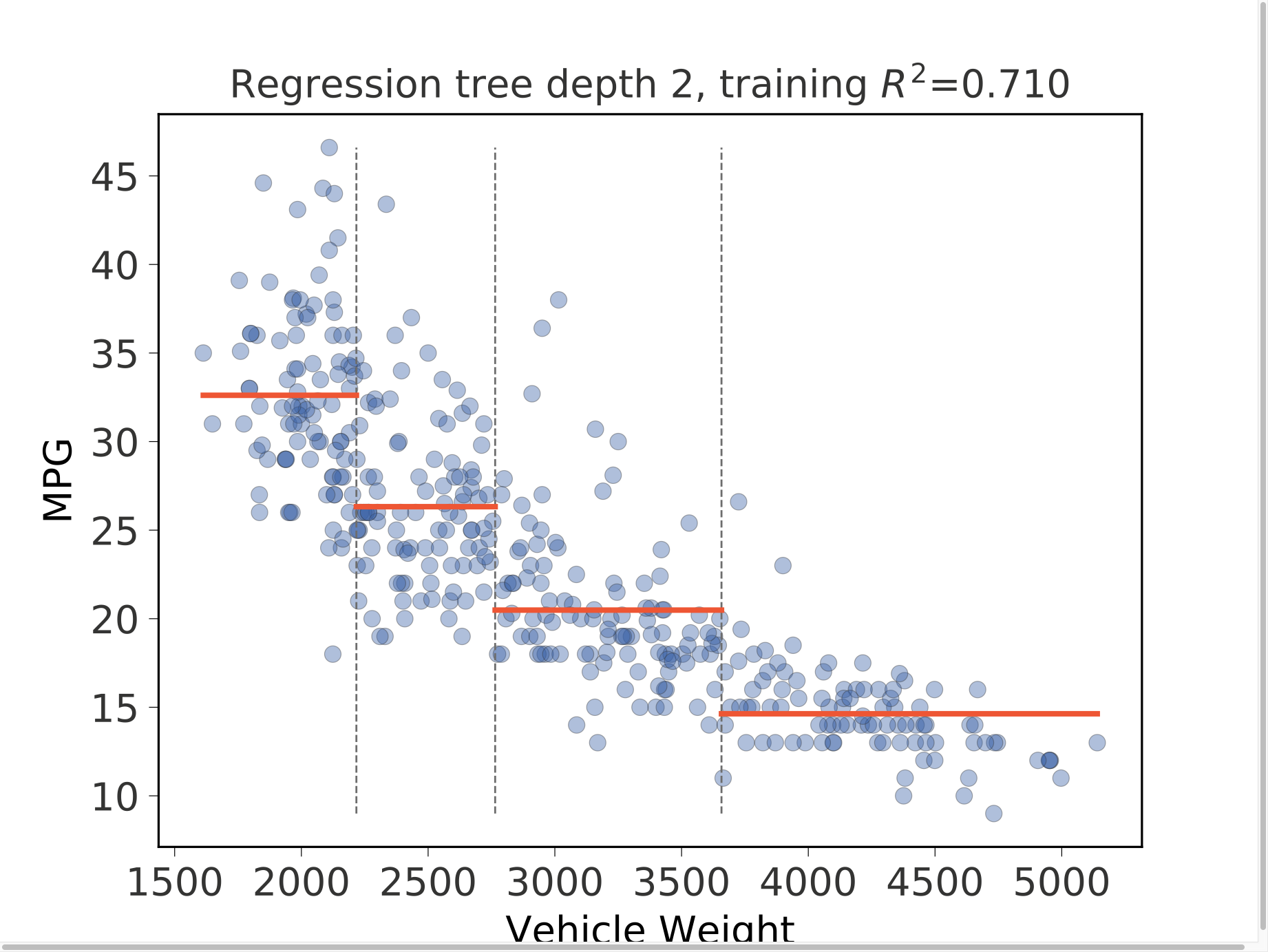import pandas as pd
from sklearn.model_selection import train_test_split
import matplotlib.pyplot as plt
from dtreeviz.trees import *

X_train, y_train = df_cars.drop('MPG', axis=1), df_cars['MPG']

fig = plt.figure()
ax = fig.gca()
t = rtreeviz_univar(ax,
X_train.WGT, y_train,
max_depth=2,
feature_name='Vehicle Weight',
target_name='MPG',
fontsize=14)
plt.show()

### Regression bivariate feature-target space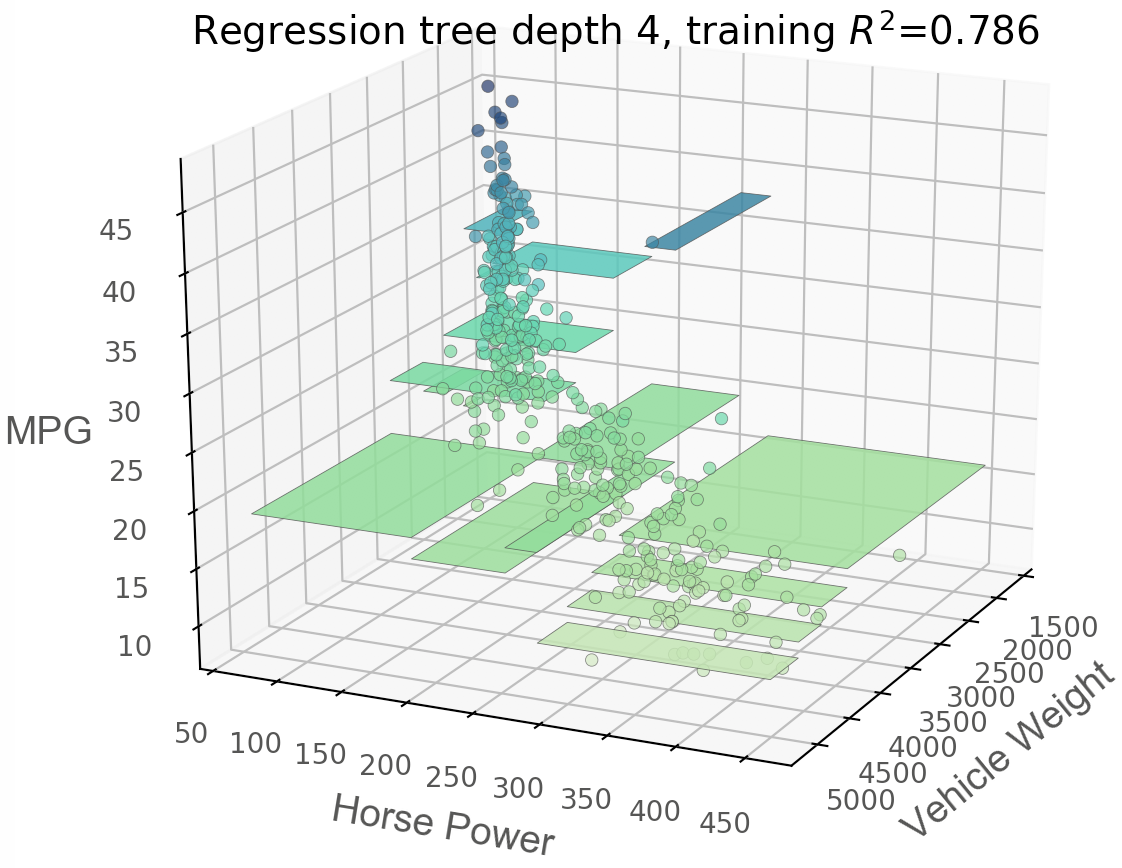from mpl_toolkits.mplot3d import Axes3D
from dtreeviz.trees import *

X = df_cars.drop('MPG', axis=1)
y = df_cars['MPG']

features = [2, 1]
X = X.values[:,features]
figsize = (6,5)
fig = plt.figure(figsize=figsize)

t = rtreeviz_bivar_3D(ax,
X, y,
max_depth=4,
feature_names=['Vehicle Weight', 'Horse Power'],
target_name='MPG',
fontsize=14,
elev=20,
azim=25,
dist=8.2,
show={'splits','title'})
plt.show()

### Regression bivariate feature-target space heatmap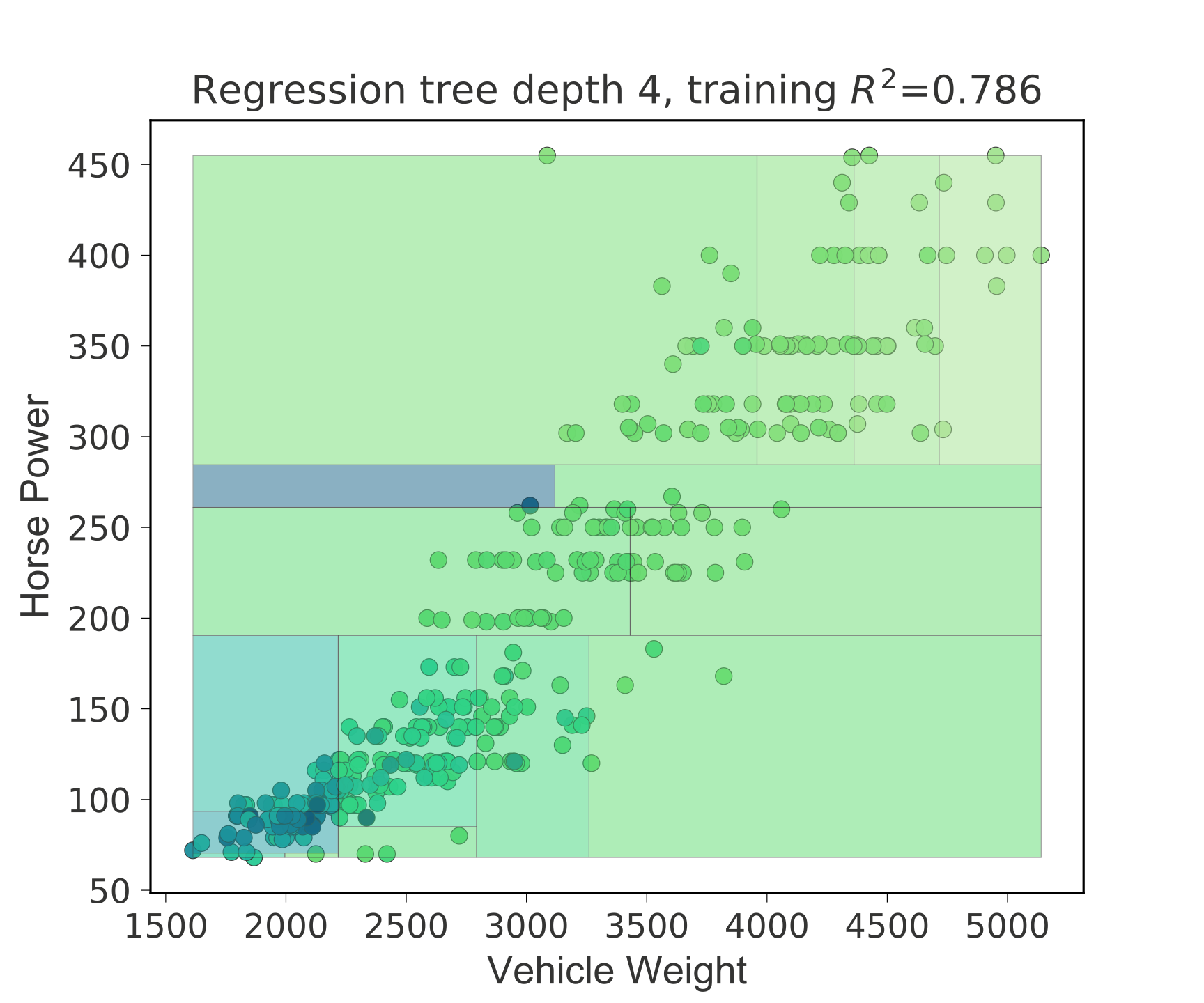from dtreeviz.trees import *

X = df_cars.drop('MPG', axis=1)
y = df_cars['MPG']

features=[2, 1]
X = X.values[:, features]
figsize = (6, 5)
fig, ax = plt.subplots(1, 1, figsize=figsize)
t = rtreeviz_bivar_heatmap(ax,
X, y,
max_depth=4,
feature_names=['Vehicle Weight', 'Horse Power'],
fontsize=14)
plt.show()

### Classification univariate feature-target space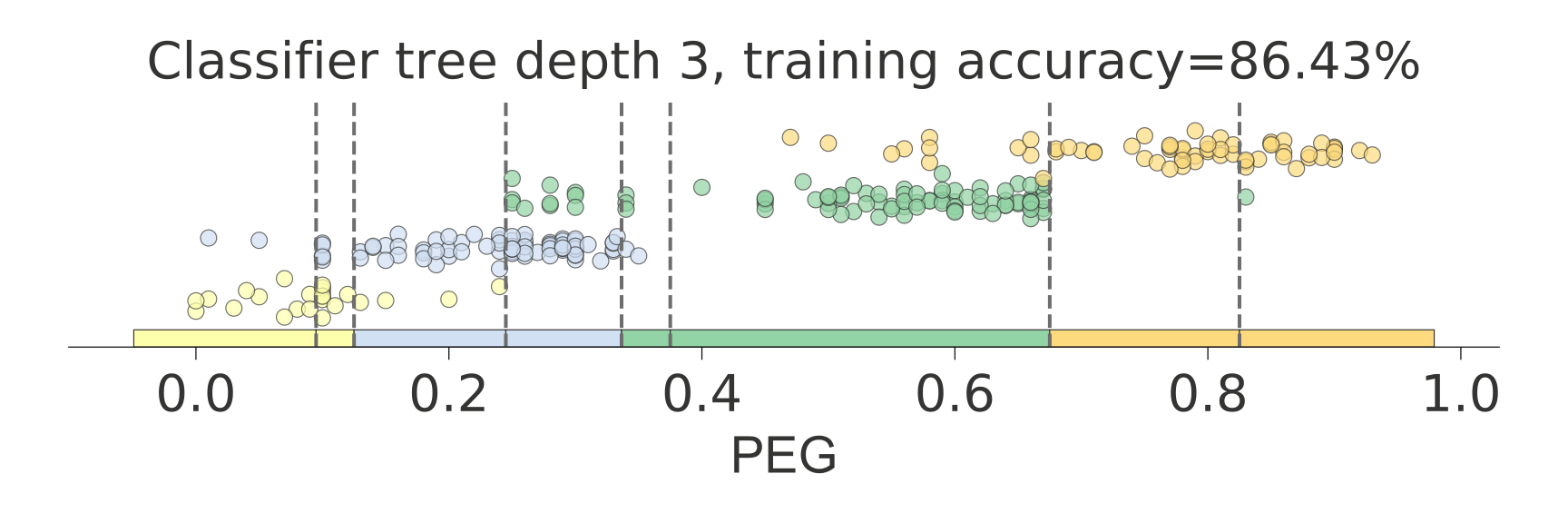from dtreeviz.trees import *

class_names = ['very_low', 'Low', 'Middle', 'High']
know['UNS'] = know['UNS'].map({n: i for i, n in enumerate(class_names)})

x_train = know.PEG
y_train = know['UNS']
figsize = (6,2)
fig, ax = plt.subplots(1, 1, figsize=figsize)
ct = ctreeviz_univar(ax, x_train, y_train, max_depth=3,
feature_name = 'PEG', class_names=class_names,
target_name='Knowledge',
nbins=40, gtype='strip',
show={'splits','title'})
plt.tight_layout()
plt.show()

### Classification bivariate feature-target space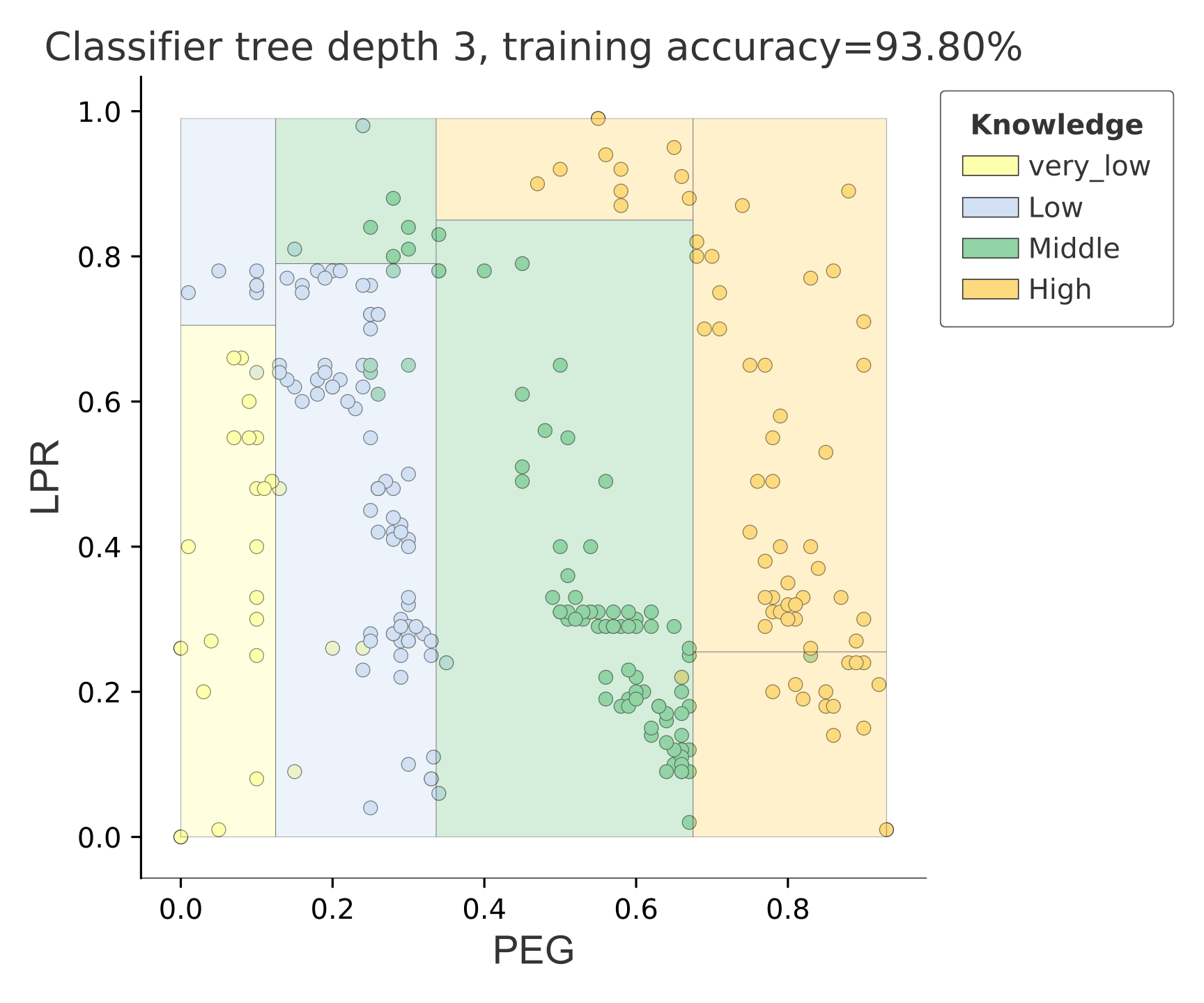from dtreeviz.trees import *

class_names = ['very_low', 'Low', 'Middle', 'High']
know['UNS'] = know['UNS'].map({n: i for i, n in enumerate(class_names)})

features=[4,3]
X_train = know.drop('UNS', axis=1)
y_train = know['UNS']
X_train = X_train.values[:, features]
figsize = (6,5)
fig, ax = plt.subplots(1, 1, figsize=figsize)
ctreeviz_bivar(ax, X_train, y_train, max_depth=3,
feature_names = ['PEG','LPR'],
class_names=class_names,
target_name='Knowledge')
plt.tight_layout()
plt.show()

## Install dtreeviz locally

Make sure to follow the install guidelines above.

To push the dtreeviz library to your local egg cache (force updates) during development, do this (from anaconda prompt on Windows):

python setup.py install -f

E.g., on Terence's box, it add /Users/parrt/anaconda3/lib/python3.6/site-packages/dtreeviz-0.3-py3.6.egg.

## Customize colors

Each function has an optional parameter colors which allows passing a dictionary of colors which is used in the plot. For an example of each parameter have a look at this notebook.

### Example

dtreeviz.trees.dtreeviz(regr,
boston.data,
boston.target,
target_name='price',
feature_names=boston.feature_names,
colors={'scatter_marker': '#00ff00'})


would paint the scatter (dots) in red.### Parameters

The colors are defined in colors.py, all options and default parameters are shown below.

COLORS = {'scatter_edge': GREY,
'scatter_marker': BLUE,
'split_line': GREY,
'mean_line': '#f46d43',
'axis_label': GREY,
'title': GREY,
'legend_title': GREY,
'legend_edge': GREY,
'edge': GREY,
'color_map_min': '#c7e9b4',
'color_map_max': '#081d58',
'classes': color_blind_friendly_colors,
'rect_edge': GREY,
'text': GREY,
'highlight': HIGHLIGHT_COLOR,
'wedge': WEDGE_COLOR,
'text_wedge': WEDGE_COLOR,
'arrow': GREY,
'node_label': GREY,
'tick_label': GREY,
'leaf_label': GREY,
'pie': GREY,
}


The color needs be in a format matplotlib can interpret, e.g. a html hex like '#eeefff' .

classes needs to be a list of lists of colors with a minimum length of your number of colors. The index is the number of classes and the list with this index needs to have the same amount of colors.